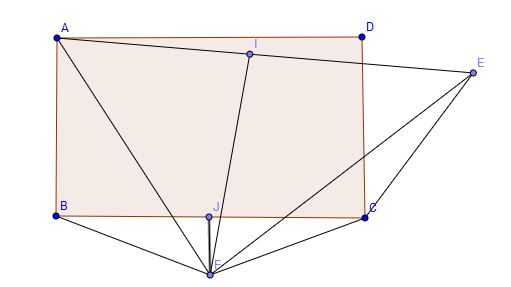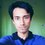# Being Equal To Something Greater Than Yourself: Another FallacyIn our previous post , we saw a mathematical fallacy and hopefully you enjoyed it. In this post we're going to see another fallacy. This one's one of my favorites because it doesn't rely on an algebraic technicality like division by zero. What makes this one work is far more devious!

The title of this post is quite thought-provoking [hopefully]. Can you be equal to something that is greater than you? No. That would be illogical. But we're going to use a loop-hole to 'prove' that. Brace yourself!Alt text

$ABCD$ is a rectangle and $CE=CD$. $FJ$ and $FI$ are the perpendicular bisectors of $BC$ and $AE$ respectively. And $J$ and $I$ are the midpoints of $BC$ and $AE$ respectively. We're going to join $F$ with $A$, $B$, $C$ and $E$ and we're ready for 'proving'!

Now, since $F$ is a point on the perpendicular bisector of $AE$,

$FA=FE$

Because of a similar argument,

$FB=FC$

And, $AB=DC$ because they're the opposite sides of a rectangle.

Notice that we've just proved that $\triangle ABF$ and $\triangle ECF$ are congruent [$\text{SSS}$ congruence].

So, $\angle ABF=\angle ECF \cdots(1)$

And also $\angle JBF=\angle JCF \cdots(2)$ because $FC=FB$.

Now subtract $(2)$ from $(1)$.

We have $\angle ABF-\angle JBF=\angle ECF-\angle JCF$

In other words, $\angle ABJ=\angle ECJ$.

But that's impossible! Because $\angle ABJ$ is a right angle and $\angle ECJ$ is an obtuse angle! $\angle ABJ$ is equal to something greater than itself. Can you spot the fallacious step?

Now's the time I tell you stop reading and think about this for a while [even though most of you don't listen :)]. So, start thinking!

Congratulations to everyone who gave this problem some time and thought! Before we find out our mistake, I want to ask you a question.

Is it necessary or compulsory to draw an accurate figure in order to do a geometric proof?

No. Actually you can't ever draw an accurate figure or diagram to be completely strict. We draw figures in our proofs as references. Figures help us follow the arguments presented in a proof. But they are not a necessity.

The figure I gave you in the beginning of the post is inaccurate. It is misleadingly inaccurate!

So, did I commit a crime?

No! We just said that it isn't compulsory to have accurate figures. It isn't wrong to draw inaccurate figures in proofs. But it is wrong to draw an inaccurate figure and make incorrect implicit assumptions from it. And that's what we did.

Everything up until the last step of this 'proof' is $100$% correct [if I haven't made any typos]. But in the last step we assumed something without proof and that led to this absurd conclusion.

When you draw the an accurate enough figure, you'll see that even though $\angle ECJ$ is an obtuse angle, it is not equal to $\angle ECF-\angle JCF$. We only assumed this from the figure [which was inaccurate]. We didn't prove it. And look what it did!

Here is a more accurate depiction of the whole situation:Alt text

Now you can see that $\angle ECF-\angle JCF\neq\angle ECJ$. Our faith in reality is restored!

If you want to check if everything else is okay, you can apply the previous arguments in the new picture. You'll see that everything works. The sides really are equal and the triangles are congruent as well. Everything works up until the last step where we assumed something unwarrantedly.

Unwarranted assumptions are really dangerous. That's why you sometimes see some kind of warning against them in some Brilliant problems [Details and assumptions: Be very careful about how you draw the picture. Don't make any unwarranted assumptions].

Can you resolve this fallacy without drawing an accurate figure? This is a good exercise for people who solve geometry problems without drawing figures [personally, I can't solve geometry problems without drawing figures]. Feel free to post your comments in the comments section below.Note by Mursalin Habib
6 years, 11 months ago

This discussion board is a place to discuss our Daily Challenges and the math and science related to those challenges. Explanations are more than just a solution — they should explain the steps and thinking strategies that you used to obtain the solution. Comments should further the discussion of math and science.

When posting on Brilliant:

• Use the emojis to react to an explanation, whether you're congratulating a job well done , or just really confused .
• Ask specific questions about the challenge or the steps in somebody's explanation. Well-posed questions can add a lot to the discussion, but posting "I don't understand!" doesn't help anyone.
• Try to contribute something new to the discussion, whether it is an extension, generalization or other idea related to the challenge.

MarkdownAppears as
*italics* or _italics_ italics
**bold** or __bold__ bold
- bulleted- list
• bulleted
• list
1. numbered2. list
1. numbered
2. list
Note: you must add a full line of space before and after lists for them to show up correctly
paragraph 1paragraph 2

paragraph 1

paragraph 2

[example link](https://brilliant.org)example link
> This is a quote
This is a quote
    # I indented these lines
# 4 spaces, and now they show
# up as a code block.

print "hello world"
# I indented these lines
# 4 spaces, and now they show
# up as a code block.

print "hello world"
MathAppears as
Remember to wrap math in $$ ... $$ or $ ... $ to ensure proper formatting.
2 \times 3 $2 \times 3$
2^{34} $2^{34}$
a_{i-1} $a_{i-1}$
\frac{2}{3} $\frac{2}{3}$
\sqrt{2} $\sqrt{2}$
\sum_{i=1}^3 $\sum_{i=1}^3$
\sin \theta $\sin \theta$
\boxed{123} $\boxed{123}$

Sort by:

Here I am with another post! Feel free to post your comments and feedback! And I hope you enjoy this!

- 6 years, 11 months ago

Extremely intriguing fallacy you have there! I never knew these types of erred proofs could go out any farther than dividing by $0$, or taking the logarithm of a negative number. Great job, again!

- 6 years, 11 months ago

Thanks!

Actually I find geometric fallacies like these more interesting than the algebraic ones saying $1=2$. Maybe this is due to the fact that geometric fallacies are a more recent discovery for me while I've been seeing division by zero 'proofs' for a long time. I have a couple more fallacies like this and I'll be posting them.

And false proofs don't have to be restricted to these as well! Here is an adaptation of a classic fallacious proof that uses flawed ideas of induction [you probably know about this one as I see you have a comment in the comments section about it].

- 6 years, 11 months ago

U hit the right spot with this post ! Awesome! U know i always disliked geometry because at the back of my mind i knew there might be such inaccuracies with my drawings this eats away at me right through my solution or proof u will agree that given long proofs which also involve lots of algebraic manipulations it becomes excedingly difficult to spot these subtleties any way out anyone?

- 6 years, 11 months ago

Nice. The original proof fails because we didn't consider the direction of the angle. In your explanation, you show how the angle is actually 'the other way', compared to what we thought the initial diagram indicated.

Have you seen the "proof" that all triangles are isosceles?

- 6 years, 11 months ago

Yes, I have. And I was thinking of posting a note about that in the near future.

- 6 years, 11 months ago

That one was pretty cool :P I saw it when I was reading this book called "the math olympian" (i think the author took it down from his site now...) The girl in the book solved it using a two-column proof.

- 6 years, 11 months ago

May I ask how did you create the diagrams?

- 6 years, 11 months ago

Geogebra.

- 6 years, 11 months ago

Looks like Geogebra.

- 6 years, 11 months ago

hello,i am madhuri i m new on brilliant... i want to ask about mathematics...how to solve integration problems.....

- 6 years ago

Check out the Calculus - Integration and Mechanics practice sections!

Staff - 6 years ago